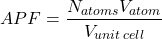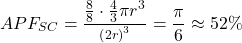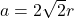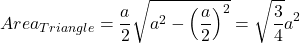# What is Atomic Packing Factor (and How to Calculate it for SC, BCC, FCC, and HCP)?

Atomic Packing Factor (APF) tells you what percent of an object is made of atoms vs empty space. You can think of this as a volume density, or as an indication of how tightly-packed the atoms are.

For quick reference, I have created a table below of atomic packing factor (APF) values ​​for common crystal structures. Later in the article, I explain step-by-step how to calculate them.

Calculating the atomic packing factor for a crystal is simple: for some repeating volume, calculate the volume of the atoms inside and divide by the total volume.Usually, this “repeating volume” is just the volume of the unit cell. The unit cell is defined as the simplest repeating unit in a crystal.

Assuming all atoms have the same size, and are arranged in a repeating crystal lattice,wheremeans number andmeans volume.

For a more complicated description of having multiple kinds of atoms, click here to expand.

If you have multiple kinds of atoms, you need to include the number and volume of each atom.whereis the volume of each type of atom. In metals there is usually only one atom, but in a ceramic, suppose there are 3 kinds of atoms:,, and. The expanded version would look like this:Additionally, atomic packing factor uses the hard sphere model. That means each atom has the volume of a sphere. Assuming the atoms are hard spheres with radiusin a cubic unit cell with lattice parameter,This might be a little hard to conceptualize, so let’s start by dropping into 1- and 2-dimensions.

### 1-Dimensional Packing Factor: Linear Density

Since we’re in 1D, formulas with volume don’t apply. Don’t worry! If you understand the concept, you won’t need a formula at all.

Remember, we want to find the space taken by atoms compared to the total space. Since we’re in 1-dimension, “space” means “length.” We can say that 1-dimensional packing is a linear density or line density.

For any particular direction, you need to draw a line and determine what percent of the line is covered by a circle.

Of course, you can’t just draw any random line. The line needs to be along the crystal “cell.”

If you’re not sure how to do this in 1-dimension, click here to expand.

This picture may make it seem like the unit cell is just an atom, but that’s only true in the close-packed direction. Usually, it makes the most sense to draw the unit cell so that a half-atom sticks out on both ends (like the red unit cell).

See, if the atoms are not “close-packed,” the unit cell will need to have more space. In other words, the lattice will be larger than the basis.

(In the arrangement above, the lattice and basis are the same size, which is why you define the unit cell around the circle with no extra space).

As long as the line you draw is a valid representation of the overall crystal symmetry, any line will work!

Simply take the length of the line covered by circles, and divide by the total length of the line.

The maximum packing factor is 1, which means 100% of the line is occupied by a circle.

If you had a packing factor larger than one, it would mean that somehow multiple circles overlapped on the same section of the line.

### 2-Dimensional Packing Factor: Planar Density

In 2 dimensions, space is area, rather than a line or a volume. Determining the packing factor works exactly the same way, however. We call this the planar density or area density.

Simply find a crystallographically repeating area (the unit cell will always work) and divide that area by the area covered by the circles.

Look at that! These two arrangements have the same packing. Any idea why that is . . . ?

Now, let’s move into 3 dimensions and take a look at how to calculate APF for the 4 common unit cells.

### Simple Cubic (SC) Lattice Length and APF

As before, we want to know how much of the crystal space is occupied by atoms vs empty space. We have entered 3-dimensions (the real world), so space is volume. To perform this calculation, we need to know the volume of a cube and the volume of a sphere.

Volume of a cube:, whereis the length of a side.

Volume of a sphere:, whereis the radius of the sphere.

Now, we have 2 variables:(radius of atom) and(side length of cube).

It turns out thatandcan be written in terms of each other.

A simple cubic (SC) unit cell is a cube with an atom on each corner of the cube. The size of the cube will be determined by the size of the atoms!

As you can see, for the simple cubic cell, the lattice parameter is just twice the radius. Now, in terms of the radius, we can say that the volume of the cube is:Each atom is a sphere, so the volume per atom is:whereis the radius of the sphere.

But, how many atoms do we have per unit cell? At first glance, it looks like there are 8 atoms: on on each corner of the cell.

However, you need to consider that when you stack unit cells to make the full crystal, each corner is shared with 8 cells. So each of the 8 atoms contributes ⅛ of its total volume. In total, there is 1 full atom per unit cell.

Now if we divide the volume of an atom by the volume of the unit cell, the atomic packing factor for a simple cubic crystal is:### Body-Centered Cubic (BCC) Lattice Length and APF

To calculate the atomic packing factor, what do we need first? The volume of the atoms, and the volume of the unit cell.

For some radius of the atoms, we can calculate their volume usingThe volume of the cube isin terms of the lattice constant, so let’s writein terms of.

In a BCC crystal, the body diagonal is the close-packed direction. I hope this is clear in the image below.

Since the body diagonal is the close-packed direction, the atoms touch each other. That means the body diagonal will be a multiple of the atomic radius. In this case, the body diagonal is.

Now, it’s time to use the pythagorean theorem (you could also use 3D vector math, but if you don’t know what that is, the trigonometry is not so complicated).

First, make a triangle with the body diagonal as the hypotenuse. This is the green line in the image above. One of your triangle legs will be the cube’s side,. The other leg will be the face diagonal. You can use any variable you like for this length; I will choose.

Using the pythagorean theorem,.

Now, let’s find. We can make another triangle from a different 3D view; this time our triangle hason the hypotenuse andfor both legs.

Again using the pythagorean theorem, we see, or.

Now we can pluginto our first pythagorean theorem.So,Now that we can write the volume of the cube in terms of the atomic radius, the rest is easy!

We only need to figure out how many atoms are in each unit cell. As in the simple cubic example, there are 8 corner atoms. Each corner atom contributes ⅛ of its volume to the unit cell, so that’s equal to 1 whole atom.

Additionally, there is an atom in the middle of the cell. Since that entire atom is inside the cell, it fully contributes its volume.

In total, there are 2 atoms in the BCC unit cell. If we divide the volume of 2 atoms by the volume of the unit cell (), we find that the atomic packing factor for a body-centered cubic crystal is:### Face-Centered Cubic (FCC) Lattice Length and APF

This should be familiar by now. Volume of the atoms divided by volume of the unit cell. Let’s get the unit cell in terms of the atomic radius!

In an FCC crystal, any face diagonal is the close-packed direction. I hope you can see this in the image below.

The face diagonal, therefore, has a length of. We can get the lattice constant in terms ofwith a simple application of the pythagorean theorem.

Draw a triangle with the face diagonal (length =) as the hypotenuse. Both legs will be.Sowill be the volume of the unit cell, so let’s figure out how many atoms are in the unit cell.

As we’ve seen several times already, there will be 8 atoms on each corner, each contributing ⅛ of its total volume to the unit cell.

Additionally, there are 6 faces, with half an atom on each face (since faces are shared between two cells, an atom on the face would contribute ½ of its volume to each cell).

In total, there are 4 atoms in the FCC unit cell. Dividing the volume of 4 atoms by the volume of the cube gives us the atomic packing factor for a face-centered cubic crystal:### Hexagonal Close-Packed (HCP) Structure and APF

Now things become tricky.

The HCP crystal structure is not cubic. Luckily, it’s still relatively easy to visualize. It’s the natural way for humans to pack spheres.

Imagine you had to pack spheres into a box. You’d probably start by making a close-packed plane on the bottom of the box. Then, you’d start the next plane by placing a sphere at one off the low points–in between 3 spheres from the bottom plane. Again, the 2nd plane can be arranged as close-packed. The 3rd plane would look exactly like the 1st plane, the 4th plane would look exactly like the 2nd plane, and so on.

This is the hexagonal close-packed lattice!

We call this unit cell “hexagonal close-packed” because it looks like hexagonal planes. In almost all cases, we use the full HCP cell.

However, it’s actually possible to define a smaller unit cell from the same atomic arrangement. This is a rhombohedral cell. Since this is the smallest unit cell possible, we call this the HCP primitive unit cell

Let’s look at the primitive unit cell, because it’s simpler so it’s easy to see the lattice parameters. Unlike a cube, there are actually 2 independant lattice parameters.

Lattice parameteris the length between 2 touching atoms (so, twice the radius).

Lattice parameteris the height of the unit cell.

By taking advantage of some trigonometry, it turns out that in an ideal HCP cell, there is a definite ratio of.

#### The Hexagonal Close-Packed c/a ratio

If you look at the central atom in the primitive cell, you can see that it has a distancebetween the atoms in the plane above and in the plane below. If you projected the atom into one of those planes, it would be exactly in the middle of 3 atoms.

This position is the center of the equilateral triangle. If you’re a big-time nerd and you already know how to calculate the centroid of an equilateral triangle, keep reading.

Otherwise, click this button.

Let’s draw a line between the center of the triangle and one of its corners. We can call this. Because the angles of an equilateral triangle are all 60°, the angle betweenandis 30°.SoNow we can make another triangle, between,, and.Which means thatOrAnd remembering that,Now that we haveand, we can calculate the volume of the hexagonal unit cell.

#### Hexagonal Close-Packed Unit Cell Volume

The hexagonal unit cell is just a hexagonal prism. You can google or memorize the answer quite easily, but in a test you might get extra points for deriving the result yourself.

Start by breaking this into parts. The volume of the hexagonal prism will be the area of the hexagon * the height of the prism. The area of the hexagon is just 6 equilateral triangles.

Let’s start by calculating the area of a single triangle. Any triangle’s area isEach side of the triangle has a length, so let’s use that as our base. Now we need to find the height of the triangle.

Once again, the pythagorean theorem saves the day! We can make right triangle between,, and the height.Which meansSo the area of the triangle isAnd since there are 6 equilateral triangles per hexagon,Multiplying this area by the height givesand usingand,Now that we know the volume of the HCP unit cell, we can calculate it’s APF!

#### Hexagonal Close-Packed Atomic Packing Fraction

The hard part is behind us. The atomic packing fraction (APF) is just the amount of atom inside the unit cell, compared to the overall size of the unit cell.

For the HCP cell, there are 12 corner atoms. Each corner atom has ⅙ of its volume inside the unit cell. To visualize this, imagine that you joined many unit cells together. Each corner atom would be shared between 6 other cells, so it contributes ⅙ to each.

There are 2 atoms on the face, which each contribute ½ of their volume.

There are 3 atoms in the center, which fully contribute their volume to the unit cell.

Altogether, that’s 6 atoms per unit cell!

We know the volume of a sphere and we already calculated the volume of the unit cell, so### Final Thoughts

That’s it! You’ve learned how to calculate the lattice parameters and atomic packing fraction for simple cubic (SC), body-centered cubic (BCC), face-centered cubic (FCC), and hexagonal close-packed (HCP) crystal systems.

Remember, APF is just the volume of the atoms within the unit cell, divided by the total volume of the unit cell. You use this to calculate the APF of any crystal system, even if it’s non-cubic or has multiple kinds of atoms!